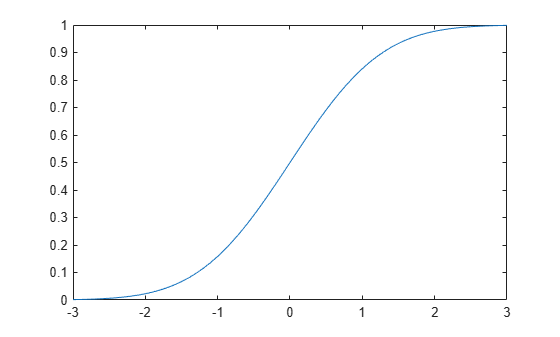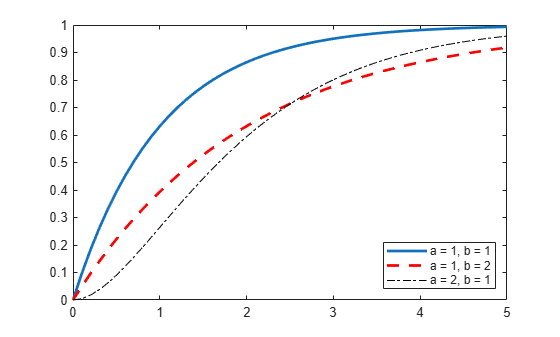Documentation

cdf

Cumulative distribution function

Description

example

y = cdf('name',x,A) returns the cumulative distribution function (cdf) for the one-parameter distribution family specified by 'name' and the distribution parameter A, evaluated at the values in x.

example

y = cdf('name',x,A,B) returns the cdf for the two-parameter distribution family specified by 'name' and the distribution parameters A and B, evaluated at the values in x.

y = cdf('name',x,A,B,C) returns the cdf for the three-parameter distribution family specified by 'name' and the distribution parameters A, B, and C, evaluated at the values in x.

y = cdf('name',x,A,B,C,D) returns the cdf for the four-parameter distribution family specified by 'name' and the distribution parameters A, B, C, and D, evaluated at the values in x.

example

y = cdf(pd,x) returns the cdf of the probability distribution object pd, evaluated at the values in x.

y = cdf(___,'upper') returns the complement of the cdf using an algorithm that more accurately computes the extreme upper-tail probabilities. 'upper' can follow any of the input arguments in the previous syntaxes.

Examples

collapse all

Create a standard normal distribution object with the mean, $\mu$, equal to 0 and the standard deviation, $\sigma$, equal to 1.

mu = 0;
sigma = 1;
pd = makedist('Normal','mu',mu,'sigma',sigma);

Define the input vector x to contain the values at which to calculate the cdf.

x = [-2,-1,0,1,2];

Compute the cdf values for the standard normal distribution at the values in x.

y = cdf(pd,x)
y = 1×5

0.0228    0.1587    0.5000    0.8413    0.9772

Each value in y corresponds to a value in the input vector x. For example, at the value x equal to 1, the corresponding cdf value y is equal to 0.8413.

Alternatively, you can compute the same cdf values without creating a probability distribution object. Use the cdf function, and specify a standard normal distribution using the same parameter values for $\mu$ and $\sigma$.

y2 = cdf('Normal',x,mu,sigma)
y2 = 1×5

0.0228    0.1587    0.5000    0.8413    0.9772

The cdf values are the same as those computed using the probability distribution object.

Create a Poisson distribution object with the rate parameter, $\lambda$, equal to 2.

lambda = 2;
pd = makedist('Poisson','lambda',lambda);

Define the input vector x to contain the values at which to calculate the cdf.

x = [0,1,2,3,4];

Compute the cdf values for the Poisson distribution at the values in x.

y = cdf(pd,x)
y = 1×5

0.1353    0.4060    0.6767    0.8571    0.9473

Each value in y corresponds to a value in the input vector x. For example, at the value x equal to 3, the corresponding cdf value y is equal to 0.8571.

Alternatively, you can compute the same cdf values without creating a probability distribution object. Use the cdf function, and specify a Poisson distribution using the same value for the rate parameter, $\lambda$.

y2 = cdf('Poisson',x,lambda)
y2 = 1×5

0.1353    0.4060    0.6767    0.8571    0.9473

The cdf values are the same as those computed using the probability distribution object.

Create a standard normal distribution object.

pd = makedist('Normal')
pd =
NormalDistribution

Normal distribution
mu = 0
sigma = 1

Specify the x values and compute the cdf.

x = -3:.1:3;
p = cdf(pd,x);

Plot the cdf of the standard normal distribution.

plot(x,p)Create three gamma distribution objects. The first uses the default parameter values. The second specifies a = 1 and b = 2. The third specifies a = 2 and b = 1.

pd_gamma = makedist('Gamma')
pd_gamma =

Gamma distribution
a = 1
b = 1

pd_12 = makedist('Gamma','a',1,'b',2)
pd_12 =

Gamma distribution
a = 1
b = 2

pd_21 = makedist('Gamma','a',2,'b',1)
pd_21 =

Gamma distribution
a = 2
b = 1

Specify the x values and compute the cdf for each distribution.

x = 0:.1:5;
cdf_gamma = cdf(pd_gamma,x);
cdf_12 = cdf(pd_12,x);
cdf_21 = cdf(pd_21,x);

Create a plot to visualize how the cdf of the gamma distribution changes when you specify different values for the shape parameters a and b.

figure;
J = plot(x,cdf_gamma);
hold on;
K = plot(x,cdf_12,'r--');
L = plot(x,cdf_21,'k-.');
set(J,'LineWidth',2);
set(K,'LineWidth',2);
legend([J K L],'a = 1, b = 1','a = 1, b = 2','a = 2, b = 1','Location','southeast');
hold off;Fit Pareto tails to a $t$ distribution at cumulative probabilities 0.1 and 0.9.

t = trnd(3,100,1);
obj = paretotails(t,0.1,0.9);
[p,q] = boundary(obj)
p = 2×1

0.1000
0.9000

q = 2×1

-1.8487
2.0766

Compute the cdf at the values in q.

cdf(obj,q)
ans = 2×1

0.1000
0.9000

Input Arguments

collapse all

Probability distribution name, specified as one of the probability distribution names in this table.

'name'DistributionInput Parameter AInput Parameter BInput Parameter CInput Parameter D
'Beta'Beta Distributiona first shape parameterb second shape parameter
'Binomial'Binomial Distributionn number of trialsp probability of success for each trial
'BirnbaumSaunders'Birnbaum-Saunders Distributionβ scale parameterγ shape parameter
'Burr'Burr Type XII Distributionα scale parameterc first shape parameterk second shape parameter
'Chisquare'Chi-Square Distributionν degrees of freedom
'Exponential'Exponential Distributionμ mean
'Extreme Value'Extreme Value Distributionμ location parameterσ scale parameter
'F'F Distributionν1 numerator degrees of freedomν2 denominator degrees of freedom
'Gamma'Gamma Distributiona shape parameterb scale parameter
'Generalized Extreme Value'Generalized Extreme Value Distributionk shape parameterσ scale parameterμ location parameter
'Generalized Pareto'Generalized Pareto Distributionk tail index (shape) parameterσ scale parameterμ threshold (location) parameter
'Geometric'Geometric Distributionp probability parameter
'HalfNormal'Half-Normal Distributionμ location parameterσ scale parameter
'Hypergeometric'Hypergeometric Distributionm size of the populationk number of items with the desired characteristic in the populationn number of samples drawn
'InverseGaussian'Inverse Gaussian Distributionμ scale parameterλ shape parameter
'Logistic'Logistic Distributionμ meanσ scale parameter
'LogLogistic'Loglogistic Distributionμ mean of logarithmic valuesσ scale parameter of logarithmic values
'Lognormal'Lognormal Distributionμ mean of logarithmic valuesσ standard deviation of logarithmic values
'Nakagami'Nakagami Distributionμ shape parameterω scale parameter
'Negative Binomial'Negative Binomial Distributionr number of successesp probability of success in a single trial
'Noncentral F'Noncentral F Distributionν1 numerator degrees of freedomν2 denominator degrees of freedomδ noncentrality parameter
'Noncentral t'Noncentral t Distributionν degrees of freedomδ noncentrality parameter
'Noncentral Chi-square'Noncentral Chi-Square Distributionν degrees of freedomδ noncentrality parameter
'Normal'Normal Distributionμ mean σ standard deviation
'Poisson'Poisson Distributionλ mean
'Rayleigh'Rayleigh Distributionb scale parameter
'Rician'Rician Distributions noncentrality parameterσ scale parameter
'Stable'Stable Distributionα first shape parameterβ second shape parameterγ scale parameterδ location parameter
'T'Student's t Distributionν degrees of freedom
'tLocationScale't Location-Scale Distributionμ location parameterσ scale parameterν shape parameter
'Uniform'Uniform Distribution (Continuous)a lower endpoint (minimum)b upper endpoint (maximum)
'Discrete Uniform'Uniform Distribution (Discrete)n maximum observable value
'Weibull'Weibull Distributiona scale parameterb shape parameter

Example: 'Normal'

Values at which to evaluate the cdf, specified as a scalar value or an array of scalar values.

If one or more of the input arguments x, A, B, C, and D are arrays, then the array sizes must be the same. In this case, cdf expands each scalar input into a constant array of the same size as the array inputs. See 'name' for the definitions of A, B, C, and D for each distribution.

Example: [0.1,0.25,0.5,0.75,0.9]

Data Types: single | double

First probability distribution parameter, specified as a scalar value or an array of scalar values.

If one or more of the input arguments x, A, B, C, and D are arrays, then the array sizes must be the same. In this case, cdf expands each scalar input into a constant array of the same size as the array inputs. See 'name' for the definitions of A, B, C, and D for each distribution.

Data Types: single | double

Second probability distribution parameter, specified as a scalar value or an array of scalar values.

If one or more of the input arguments x, A, B, C, and D are arrays, then the array sizes must be the same. In this case, cdf expands each scalar input into a constant array of the same size as the array inputs. See 'name' for the definitions of A, B, C, and D for each distribution.

Data Types: single | double

Third probability distribution parameter, specified as a scalar value or an array of scalar values.

If one or more of the input arguments x, A, B, C, and D are arrays, then the array sizes must be the same. In this case, cdf expands each scalar input into a constant array of the same size as the array inputs. See 'name' for the definitions of A, B, C, and D for each distribution.

Data Types: single | double

Fourth probability distribution parameter, specified as a scalar value or an array of scalar values.

If one or more of the input arguments x, A, B, C, and D are arrays, then the array sizes must be the same. In this case, cdf expands each scalar input into a constant array of the same size as the array inputs. See 'name' for the definitions of A, B, C, and D for each distribution.

Data Types: single | double

Probability distribution, specified as a probability distribution object created with a function or app in this table.

Function or AppDescription
makedistCreate a probability distribution object using specified parameter values.
fitdistFit a probability distribution object to sample data.
Distribution FitterFit a probability distribution to sample data using the interactive Distribution Fitter app and export the fitted object to the workspace.
paretotailsCreate a piecewise distribution object that has generalized Pareto distributions in the tails.

Output Arguments

collapse all

cdf values, returned as a scalar value or an array of scalar values. y is the same size as x after any necessary scalar expansion. Each element in y is the cdf value of the distribution, specified by the corresponding elements in the distribution parameters (A, B, C, and D) or the probability distribution object (pd), evaluated at the corresponding element in x.

Alternative Functionality

• cdf is a generic function that accepts either a distribution by its name 'name' or a probability distribution object pd. It is faster to use a distribution-specific function, such as normcdf for the normal distribution and binocdf for the binomial distribution. For a list of distribution-specific functions, see Supported Distributions.

• Use the Probability Distribution Function app to create an interactive plot of the cumulative distribution function (cdf) or probability density function (pdf) for a probability distribution.